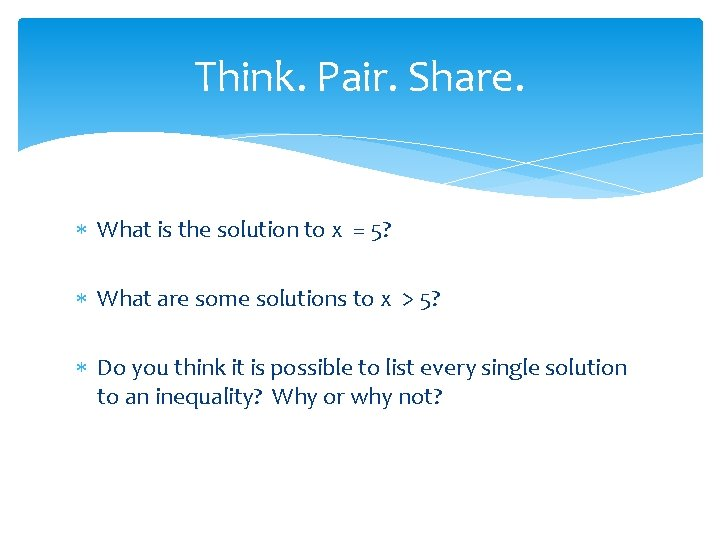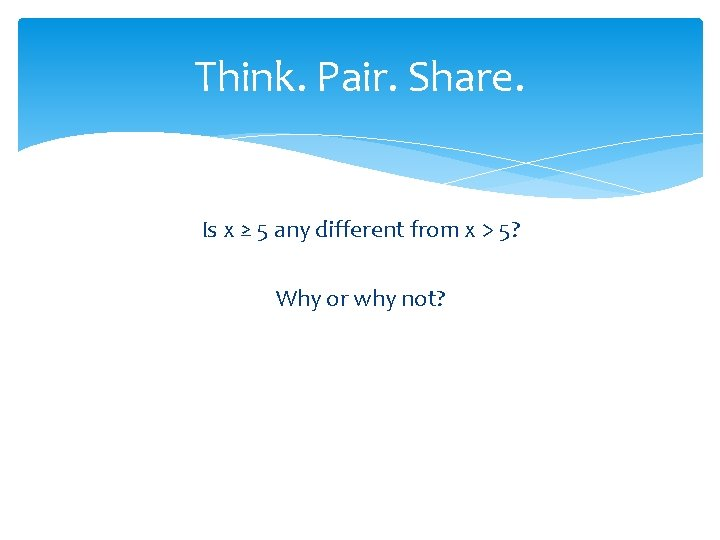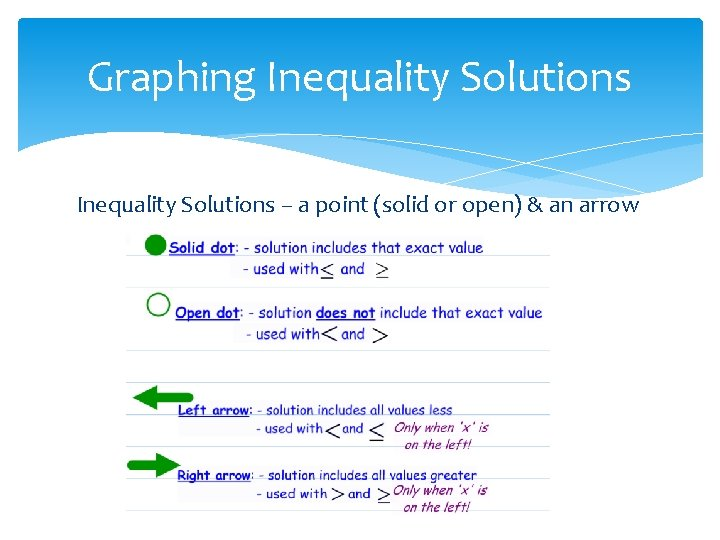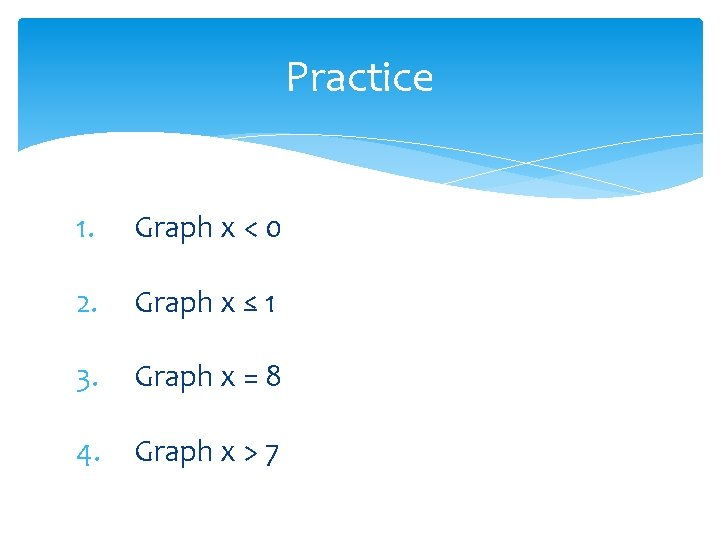# Equations and Inequalities Part 5 Writing Graphing Inequalities

• Slides: 10Equations and Inequalities Part 5: Writing & Graphing Inequalities Essential Question: How does the solution to an equation relate to the solutions of an inequality of the same nature? How can I represent the solution to an equation or inequality on a number line (graph)?Think. Pair. Share. What is the solution to x = 5? What are some solutions to x > 5? Do you think it is possible to list every single solution to an inequality? Why or why not?Think. Pair. Share. Is x ≥ 5 any different from x > 5? Why or why not?Graphing You can represent the solution to an equation or an inequality by graphing it on a number line. X=2 X>2Graphing Equation Solutions – a point (a solid dot) A solid circle states that the solution is the dot’s valueGraphing Inequality Solutions – a point (solid or open) & an arrowExamples of Inequality SolutionsPractice 1. Graph x < 0 2. Graph x ≤ 1 3. Graph x = 8 4. Graph x > 7Write and Graph an Inequality to represent the situation 1. Tommy (t) has at least \$52 2. No more than 300 students (s) can fit in the cafeteria 3. Sue has more than 5 pencils (p) 4. Abby has less than \$12Practice & Other Resources Holt Course 1 Chapter 2 Resource Book or Online Textbook (pages 7677) http: //www. coolmath-games. com/0 greaterthansudoku/index. html http: //teachers. henrico. k 12. va. us/math/hcpsalgebra 1/ module 4 -1. html